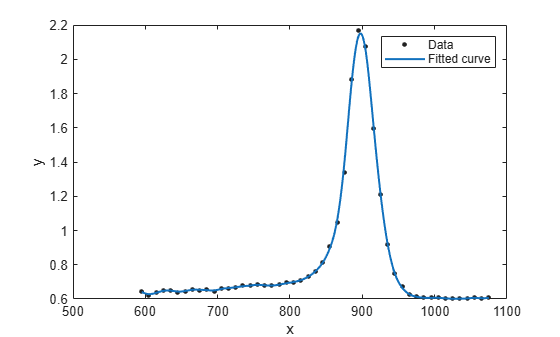Documentation

# prepareCurveData

Prepare data inputs for curve fitting

## Syntax

``````[XOut,YOut] = prepareCurveData(XIn,YIn)``````
``````[XOut,YOut,WOut] = prepareCurveData(XIn,YIn,WIn)``````

## Description

example

``````[XOut,YOut] = prepareCurveData(XIn,YIn)``` transforms data, if necessary, for curve fitting with the `fit` function. The `prepareCurveData` function transforms data as follows: Return data as columns regardless of the input shapes. Error if the number of elements do not match. Warn if the number of elements match, but the sizes differ.Convert complex to real (remove imaginary parts) and warn of this conversion.Remove `NaN` or `Inf` from data and warn of this removal.Convert nondouble to double and warn of this conversion. Specify `XIn` as empty if you want to fit curves to y data against the index. If `XIn` is empty, then `XOut` is a vector of indices into `YOut`. The `fit` function can use the vector `XOut` for the x data when there is only y data.```
``````[XOut,YOut,WOut] = prepareCurveData(XIn,YIn,WIn)``` transforms data including weights (`WIn`) for curve fitting with the `fit` function.When you generate code from the Curve Fitting app, the generated code includes a call to `prepareCurveData` (or `prepareSurfaceData` for surface fits). You can call the generated file from the command line with your original data or new data as input arguments, to recreate your fits and plots. If you call the generated file with new data, the `prepareCurveData` function ensures you can use any data that you can fit in the Curve Fitting app, by reshaping if necessary to column doubles and removing `NaN`s, `Inf`s, or the imaginary parts of complex numbers.```

## Examples

collapse all

The following commands load the example `titanium` data in which `x` and `y` are row vectors. Attempting to use rows as inputs to the `fit` function produces an error. The `prepareCurveData` function reshapes `x` and `y` to columns for use with the `fit` function.

```[x,y] = titanium(); [x,y] = prepareCurveData(x,y);```

Create and plot a fit using the reshaped data.

```f = fit(x,y,'smoothingspline'); plot(f,x,y)```## Input Arguments

collapse all

X data variable for curve fitting, specified as a scalar, a vector or a matrix. `XIn` can be empty. Specify empty (```[ ]```) when you want to fit curves to y data against index (x=1:length( y )). See `YOut`.

Data Types: `double`

Y data variable for curve fitting, specified as a scalar, a vector or a matrix.

Data Types: `double`

Weights variable for curve fitting specified, as a scalar, a vector or a matrix.

Data Types: `double`

## Output Arguments

collapse all

X data column variable prepared for curve fitting, returned as a vector.

If `XIn` is empty, then `XOut` is a vector of indices into `YOut`. The `fit` function can use the vector `XOut` for the x data when there is only y data.

Data Types: `double`

Y data column variable prepared for curve fitting, returned as a vector.

Data Types: `double`

Weights column variable prepared for curve fitting, returned as a vector.

Data Types: `double`